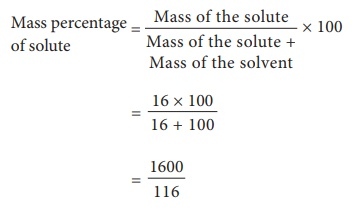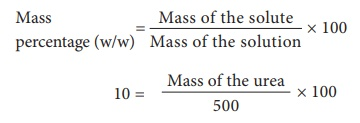Home | | Science 10th Std | Problems Based on Solubility and Percentage by Mass and Volume

# Problems Based on Solubility and Percentage by Mass and Volume

I. Problems based on solubility II. Problem based on mass percentage

Problems Based on Solubility and Percentage by Mass and Volume

## I. Problems based on solubility

1) 1.5 g of solute is dissolved in 15 g of water to form a saturated solution at 298K. Find out the solubility of the solute at the temperature.

Mass of the solvent = 15 g

Solubility of the solute  = [ Mass of the solute/ Mass of the solvent]  × 100

Solubility of the solute = [1.5/15] × 100

= 10 g

2) Find the mass of potassium chloride would be needed to form a saturated solution in 60 g of water at 303 K? Given that solubility of the KCl is 37/100 g at this temperature.

Mass of potassium chloride in 100 g of water in saturated solution = 37 g

Mass of potassium chloride in 60 g of water in saturated solution= 37/100 × 60

= 22.2 g

3) What is the mass of sodium chloride that would be needed to form a saturated solution in 50 g of water at 30°C. Solubility of sodium chloride is 36 g at 30°C?

At 30°C, 36 g of sodium chloride is dissolved in 100 g of water.

Mass of sodium chloride that would be need for 100 g of water = 36 g

Mass of sodium chloride dissolved in 50 g of water = [36 × 50] x 100

= 18 g

4) The Solubility of sodium nitrate at 50°C and 30°C is 114 g and 96 g respectively. Find the amount of salt that will be thrown out when a saturated solution of sodium nitrate containing 50 g of water is cooled from 50°C to 30°C?

Amount of sodium nitrate dissolved in 100 g of water at 50°C is 114 g

Amount of sodium nitrate  dissolving in 50 g of water at 50°C is = [114 × 50] / 100

= 57 g

Similarly amount of sodium nitrate dissolving in 50g of water at 30°C is = [96 × 50] × 100

= 48g

Amount of sodium nitrate thrown when 50g of water is cooled from 50°C to 30°C is

57 – 48 = 9 g

## II. Problem based on mass percentage

1) A solution was prepared by dissolving 25 g of sugar in 100 g of water. Calculate the mass percentage of solute.

Mass of the solute = 25 g

Mass of the solvent = 100 g2) 16 grams of NaOH is dissolved in 100 grams of water at 25°C to form a saturated solution. Find the mass percentage of solute and solvent.

Mass of the solute (NaOH) = 16 g

Mass of the solvent H2O = 100 g

(i) Mass percentage of the solute

Mass percentage of solute  = [Mass of the solute / Mass of the solute + Mass of the solvent] ×  100Mass percentage of the solute = 13.79 %

(ii) Mass percentage of solvent

= 100  - (Mass percentage of the solute)

= 100 – 13.79 = 86.21%

3) Find the amount of urea which is to be dissolved in water to get 500 g of 10% w/w aqueous solution?Mass of urea = 50g

(iii) Problem based on Volume – volume percentage.

1) A solution is made from 35 ml of Methanol and 65 ml of water. Calculate the volume percentage.

Volume of the ethanol = 35 ml

Volume of the water = 65 mlVolume percentage = [ 35 / 35+65 ]× 100

Volume percentage = [ 35/100 ] × 100

= 35%

2) Calculate the volume of ethanol in 200 ml solution of 20% v/v aqueous solution of ethanol.

Volume of aqueous solution = 200 ml

Volume percentage = 20%

Volume percentage  = [Volume of solute / Volume of solution]  × 100

20 = [Volume of ethanol/200 ] × 100

Volume of ethanol = [20 × 200] / 100 = 40 ml

Study Material, Lecturing Notes, Assignment, Reference, Wiki description explanation, brief detail
10th Science : Chapter 9 : Solutions : Problems Based on Solubility and Percentage by Mass and Volume |